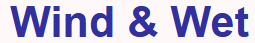# Wind turbine power calculator

The graph shows the power available from a wind turbine across a range of wind speeds. Enter the specification of your turbine in the form and see how much power it is possible to generate.
 Blade radius Blade radius in metres Tip speed ratio Used to calculate RPM Coefficient of performance Max value is the Betz limit 0.59 (16/27) Air density kg/m3

The power from the wind turbine for a given wind speed is calculated using the equation:• The maximum theoretical coefficient of performance or Betz limit is defined as 16/27 or 0.59 although in practice this would not be achievable and a lower value should be used. The coefficient of performance will typically vary with wind speed. An efficient horizontal axis wind turbine might achieve a value of 0.35. Some wind turbine efficiency and power output graphs can be found on:
• At 0°C and 100KPa the air density is 1.2754 kg/m3 but this will vary with altitude and temperature
• The tip speed ratio is included in the calculation so the rotor and alternator speed can be calculated.